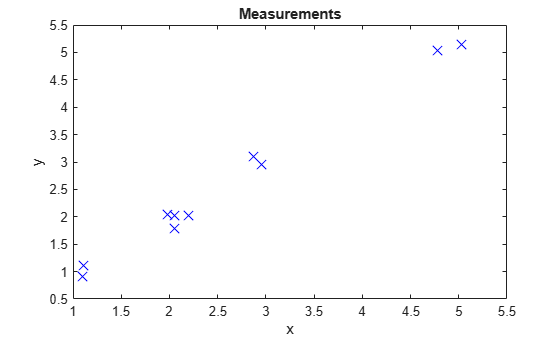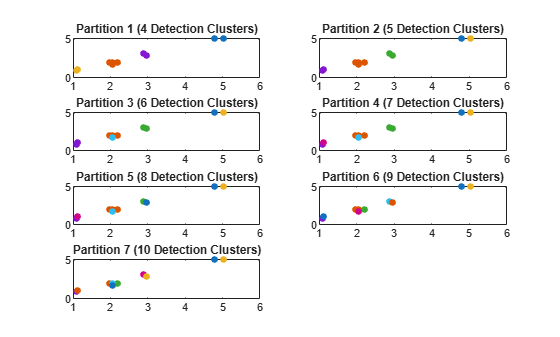# partitionDetections

Partition detections based on Mahalanobis distance

## Syntax

``partitions = partitionDetections(detections)``
``partitions = partitionDetections(detections,tLower,tUpper)``
``partitions = partitionDetections(detections,tLower,tUpper,'MaxNumPartitions',maxNumber)``
``partitions = partitionDetections(detections,allThresholds)``

## Description

Using multiple distance thresholds, the function separates detections into different detection cells based on their relative Mahalanobis distances and reports all the possible partitions. A partition of a set of detections is defined as a division of these detections into nonempty mutually exclusive detection cells. A detection cell is a group of detections whose distance to at least one other detection in the cell is less than the distance threshold. In other words, two detections belong to the same detection cell if their distance is less than the distance threshold.

example

````partitions = partitionDetections(detections)` returns possible `partitions` from `detections`, using distance partitioning algorithm. By default, the function considers all real value Mahalanobis distance thresholds between 0.5 and 6.25. ```
````partitions = partitionDetections(detections,tLower,tUpper)` allows you to specify the lower and upper bounds of the distance thresholds, `tLower` and `tUpper`.```
````partitions = partitionDetections(detections,tLower,tUpper,'MaxNumPartitions',maxNumber)` allows you to specify the maximum number of allowed partitions, `maxNumber`, in addition to the lower and upper bounds of the distance thresholds, `tLower` and `tUpper`.```
````partitions = partitionDetections(detections,allThresholds)` allows you to specify the exact thresholds considered for partition.```

## Examples

collapse all

Generate 2-D detections using `objectDetection`.

```rng(2018); % For reproducible results detections = cell(10,1); for i = 1:numel(detections) id = randi([1 5]); detections{i} = objectDetection(0,[id;id] + 0.1*randn(2,1)); detections{i}.MeasurementNoise = 0.01*eye(2); end```

Extract and display generated position measurements.

```d = [detections{:}]; measurements = [d.Measurement]; figure() plot(measurements(1,:),measurements(2,:),'x','MarkerSize',10,'MarkerEdgeColor','b') title('Measurements') xlabel('x') ylabel('y')```Generate partitions from the detections and count the number of partitions.

```partitions = partitionDetections(detections); numPartitions = size(partitions,2);```

Visualize the partitions. Each color represents a detection cell.

```figure() for i = 1:numPartitions numCells = max(partitions(:,i)); subplot(3,ceil(numPartitions/3),i); for k = 1:numCells ids = partitions(:,i) == k; plot(measurements(1,ids),measurements(2,ids),'.','MarkerSize',15); hold on; end title(['Partition ',num2str(i),' (',num2str(k),' Detection cells)']); end```## Input Arguments

collapse all

Object detections, specified as an N-element cell array of `objectDetection` objects, where N is the number of detections. You can create `detections` directly, or you can obtain `detections` from the outputs of sensor objects, such as `fusionRadarSensor`, `irSensor`, and `sonarSensor`.

Data Types: `cell`

Lower bound of distance thresholds, specified as a scalar. This argument sets the lower bound of the Mahalanobis distance thresholds considered for partition.

Example: `0.05`

Data Types: `double`

Upper bound of distance thresholds, specified as a scalar. This argument sets the upper bound of the Mahalanobis distance thresholds considered for partition.

Example: `0.98`

Data Types: `double`

Maximum number of allowed partitions, specified as a positive integer.

Example: `20`

Data Types: `double`

All thresholds for partitions, specified as an M element vector. The function calculates partitions based on each threshold value provided in `allThresholds`. Note that multiple thresholds can result in the same partition, and the function output `partitions`, given as an N-by-Q matrix with QM, only contains unique partitions.

Example: `[0.1;0.2;0.35;0.4]`

Data Types: `double`

## Output Arguments

collapse all

Partitions of detections, specified as an N-by-Q matrix. N is the number of detections, and Q is the number of partitions. Each column of the matrix represents a valid partition. In each column, the value of the ith element represents the identity number of the detection cell that the ith detection belongs to. For example, given a partition matrix P, if P(i,j) = k, then in partition j, detection i belongs to detection cell k.

 Granstorm, K., C. Lundquiest, and O. Orguner. " Extended target tracking using a Gaussian-mixture PHD filter." IEEE Transactions on Aerospace and Electronic Systems. Vol. 48, Number 4, 2012, pp. 3268–3286.